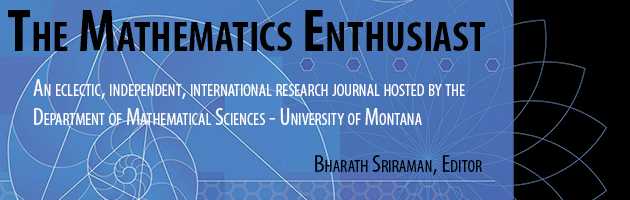•
•21

1-2

#### Abstract

Figured numbers are sequences of natural numbers that, when represented by dots, form geometric configurations. The immediate sequence of figured numbers presents in its configuration the equilateral triangles, being the basis for finding several others, as long as a relationship between them is discovered. This article shows the construction of polygonal, stellar and three-dimensional figured numbers that can be constructed using the GeoGebra software as a resource to visualize the properties that relate these numbers to the triangular numbers. It is a way of observing the relationship that the figures shown have to each other, how we can understand the structure of these sequences, when presented in the form of figures, and the possibility of building figures that represent some of the most known sequences within the number’s figures such as 2D figured numbers such as square, pentagonal, centered triangular and star numbers within 3D figured numbers. Thus, this work presents such construction through the properties of pyramidal numbers, cubic numbers, octahedral numbers and two forms of construction of the stella octangula numbers, with emphasis on the use of triangular numbers or using the other sequences that depart from this one. Furthermore, the work shows the possibility of constructing other sequences as well as the discovery of possible properties that relate them.

203

224

#### Digital Object Identifier (DOI)

10.54870/1551-3440.1624

#### Publisher

University of Montana, Maureen and Mike Mansfield Library

COinS